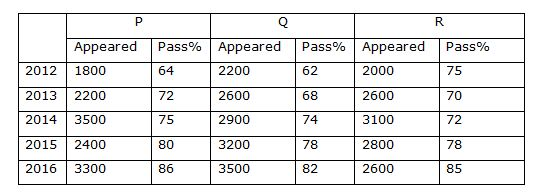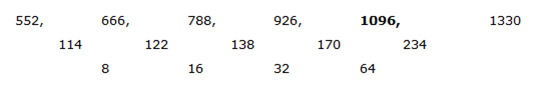# “20-20” Quantitative Aptitude | Crack IBPS RRB Assistant Prelims 2018 Day-153

Dear Readers, Find the aptitude test questions to crack latest bank exams. We regularly provide 20 aptitude test questions daily for students. Aspirants practice these questions on a regular basis to improve your score in aptitude section. Aspirants preparing for the exams can make use of this “20-20” Quantitative Aptitude Questions. Here we have started New Series of Practice Materials specially for IBPS RRB Assistant Prelims 2018. Aspirants those who are preparing for the exams can use this “20-20” Quantitative Aptitude Questions.

“20-20” Aptitude Questions | Crack IBPS RRB Assistant Prelims 2018 Day-153

Click “Start Quiz” to attend these Questions and view Solutions

Directions (1 – 5): What value should come in the place of question mark (?) in the following questions?

1) (4 × 4)3 ÷ (512 ÷ 8)4 × (32 × 8)4 = (4)? + 4

a) 8

b) 5

c) 7

d) 6

e) None of these

2) 76 % of 1285 – 35 % of 1256 =?

a) 537

b) 629

c) 451

d) 787

e) None of these

3) (2/7) of 5033 + 78 % of 650 = (?)2 + 181

a) 48

b) 46

c) 42

d) 39

e) None of these

4) 32 % of 480 + (5/7) of 1890 – 28 % of 820 =?

a) 1336

b) 1178

c) 1452

d) 1274

e) None of these

5) ? *100/(140 × 8 – 680) = 330 + 452

a) 9857

b) 10362

c) 11238

d) 8564

e) None of these

Directions (6 – 10): What value should come in the place of question mark (?) in the following number series?

6) 14, 8, 16, 59, 300, ?

a) 1486

b) 1652

c) 1893

d) 2177

e) 2525

7) 8, 17, 70, 423, 3388, ?

a) 33885

b) 25640

c) 21373

d) 29787

e) 35662

8) 14224, 7113, 2374, 598.5, ?

a) 178.3

b) 185.4

c) 126.7

d) 132.5

e) 154.6

9) 53, 26, 27, 39, 80, 197.5, ?

a) 378

b) 424

c) 517.5

d) 595.5

e) 492.5

10) 552, 666, 788, 926, ?, 1330

a) 1124

b) 1096

c) 1078

d) 1212

e) 1250

11) The ratio of age of P, 4 years ago and the age of Q, 3 years after is 3: 5. Sum of the present ages of P and Q is 49 years. Find the age of P, 3 years after?

a) 25 years

b) 28 years

c) 32 years

d) 30 years

e) None of these

12) A box contains 5 white balls, 6 violet balls and 3 yellow balls. If 2 balls are drawn randomly, then the probability of getting both the balls is either white or violet balls?

a) 11/56

b) 23/72

c) 35/83

d) 25/91

e) None of these

13) The length of train A is 150 m and that of train B is 200 m. These two trains are running in the opposite direction with the velocities of 50 km/hr and 40 km/hr respectively. Find the time taken to cross each other?

a) 14 sec

b) 21 sec

c) 18 sec

d) 25 sec

e) None of these

14) Agila, Manoj and Prithika enter into a partnership. Agila invests 2 times as much as Manoj’s investment and Manoj invests 4/7th of Prithika’s investment. If the total profit earned at the end of the year is Rs. 123500, then find the share of Manoj?

a) Rs. 28000

b) Rs. 22000

c) Rs. 26000

d) Rs. 30000

e) None of these

15) The CI on a certain sum of money for 3 years at 5 % per annum is Rs. 7881.25. Then find the principle?

a) Rs. 45000

b) Rs. 50000

c) Rs. 42000

d) Rs. 36000

e) None of these

Directions (16 – 20): Study the following information carefully and answer the given questions:

Following table shows the total no students appeared for 10th Public examination and pass %, from different schools in different years.16) Find the ratio between the total number of passing students from all the given schools together in the year 2013 to that of 2015?

a) 124: 175

b) 256: 313

c) 431: 550

d) 378: 415

e) None of these

17) Total number of appeared students in the year 2012 from School P and R together is approximately what percentage of total number of appeared students in the year 2016 from School Q and R together?

a) 62 %

b) 80 %

c) 50 %

d) 90 %

e) 100 %

18) Find the average number of passed students from School Q in the year 2012, 2014, 2015 and 2016 together?

a) 2527

b) 2413

c) 2355

d) 2219

e) None of these

19) Find the difference between the total number of appeared students in the year 2013 and 2014 together to that of total number of appeared students in the year 2015 and 2016 together from all the given schools together?

a) 750

b) 900

c) 800

d) 650

e) None of these

20) Find the total number of failed students from School P in all the given years together?

a) 2178

b) 2564

c) 3081

d) 2893

e) None of these

Direction (1-5)

46 ÷ (512/8)4 × (32 × 8)4 = (4)x + 4

46 ÷ 644 × 2564 = (4)x + 4

46 ÷ 412 × 416 = 4x + 4

46 – 12 + 16 = 4x + 4

410 = 4x + 4

x + 4 = 10

x = 6

(76/100)*1285 – (35/100)*1256 = X

X = 976.6 – 439.6

X = 537

(2/7)*5033 + (78/100)*650 = x2 + 181

1438 + 507 – 181 = x2

X2 = 1764

X = 42

(32/100)*480 + (5/7)*1890 – (28/100)*820 = x

X = 153.6 + 1350 – 229.6

X = 1274

(x*100)/(1120 – 680) = 330 + 2025

x/100)*(440) = 2355

x = 2355*(440/100)

x = 10362

Direction (6-10)

The pattern is, *0.5 + 13, *1 + 23, *2 + 33, *4 +43, *8 + 53,..

The pattern is, *2 + 1, *4 + 2, *6 +3, *8 + 4, *10 + 5,..

The pattern is, ÷ 2 +1, ÷ 3 +3, ÷ 4 +5, ÷ 5 +7,..

The pattern is, *0.5 – 0.5, *1 + 1, *1.5 -1.5, *2 + 2, *2.5 – 2.5, *3 + 3,..The difference of difference is, ×2

The ratio of ages of P, 4 years ago and the ages of Q, 3 years after = 3: 5 (3x, 5x)

Present ages of P and Q = 3x + 4, 5x – 3

According to the question,

3x + 4 + 5x – 3 = 49

8x + 1 = 49

8x = 48

X = 6

Present age of P = 3x + 4 = 22

The age of P, 3 years after = 25 years

Total probability n(S) = 14C2

Required probability n(E) = 5C2 or 6C2

P(E) = n(E)/n(S) = 5C2 or 6C2 / 14C2

= > 25/91

T = D/S

T = (150 + 200)/[(50 + 40)*(5/18)]

T = (350*18)/(90*5)

T = 14 sec

The investment of Agila and Manoj = 2: 1

Agila’s investment = (4/7)* Prithika’s investment

The investment of Manoj and Prithika = 4: 7

The investment of Agila, Manoj and Prithika = 8: 4: 7

Here, the period is not given. So,

The ratio of investment = The ratio of profit

Total profit = Rs. 123500

19’s = 123500

1’s = 6500

The share of Manoj = 4’s = Rs. 26000

C.I = P*[(1 + (r/100))3 – 1]

7881.25 = P*[(1 + 5/100)3 – 1]

7881.25 = P*[(105/100)3 – 1]

7881.25 = P*[(21/20)3 – 1]

7881.25 = P*[(9261/8000) – 1]

7881.25 = P*(1261/8000)

7881.25*(8000/1261) = P

P = Rs. 50000

Direction (16-20)

The total number of passing students from all the given schools together in the year 2013

= > 2200*(72/100) + 2600*(68/100) + 2600*(70/100)

= > 1584 + 1768 + 1820 = 5172

The total number of passing students from all the given schools together in the year 2015

= > 2400*(80/100) + 3200*(78/100) + 2800*(78/100)

= > 1920 + 2496 + 2184 = 6600

Required ratio = 5172: 6600 = 431: 550

Total number of appeared students in the year 2012 from School P and R together

= > 1800 + 2000 = 3800

Total number of appeared students in the year 2016 from School Q and R together

= > 3500 + 2600 = 6100

Required % = (3800/6100)*100 = 62 % = 62 %

The total number of passed students from School Q in the year 2012, 2014, 2015 and 2016 together

= > 2200*(62/100) + 2900*(74/100) + 3200*(78/100) + 3500*(82/100)

= > 1364 + 2146 + 2496 + 2870 = 8876

Required average = 8876/4 = 2219

The total number of appeared students in the year 2013 and 2014 together from all the given schools together

= > 2200 + 2600 + 2600 + 3500 + 2900 + 3100

= > 16900

The total number of appeared students in the year 2015 and 2016 together from all the given schools together

= > 2400 + 3200 + 2800 + 3300 + 3500 + 2600

= > 17800

Required difference = 17800 – 16900 = 900

The total number of failed students from School P in all the given years together

= > 1800*(36/100) + 2200*(28/100) + 3500*(25/100) + 2400*(20/100) + 3300*(14/100)

= > 648 + 616 + 875 + 480 + 462

= > 3081

Daily Practice Test Schedule | Good Luck

 Topic Daily Publishing Time Daily News Papers & Editorials 8.00 AM Current Affairs Quiz 9.00 AM Quantitative Aptitude “20-20” 11.00 AM Vocabulary (Based on The Hindu) 12.00 PM General Awareness “20-20” 1.00 PM English Language “20-20” 2.00 PM Reasoning Puzzles & Seating 4.00 PM Daily Current Affairs Updates 5.00 PM Data Interpretation / Application Sums (Topic Wise) 6.00 PM Reasoning Ability “20-20” 7.00 PM English Language (New Pattern Questions) 8.00 PM

IBPSGuide Recommends Affairs Cloud Current affairs PDF